## 3.2.1 Net Charge Exchange Model

The secondary electron yield is defined as the average number of electrons ejected per incident atom. The secondary electron yield was calculated from probe current measurements. Ideally this yield would be measured using neutral atoms as primary beam.

Unfortunately the ion source in ILENA only provides molecular ions. This means that all the different dissociation and ionization possibilities at the surface have to be considered separately. From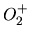impinging on MgO (see Chapter 5.5) and BaZrO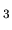the model shown in Figure 3.1 was derived.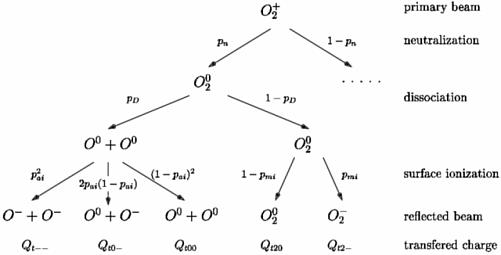Only observed charge and particle states were considered. On the incoming trajectory virtually all molecules are neutralized in front of the surface with the probability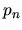, the remaining positive charged fraction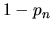is less than 0.5% - at least for MgO. This almost complete neutralization also holds for metal surfaces . Also on the incoming trajectory the now neutralized molecules dissociate with the probability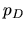. The dissociation is probably associated with the neutralization process. A molecule may capture an electron from the surface into an antibonding state and subsequently dissociate. However, dissociation was also observed for neutral primary molecules, a case not covered by this model. On the outgoing trajectory the partly dissociated primary particles get negatively ionized with a probability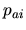for neutral atoms and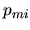for neutral molecules. For low-work-function surfaces the negative ion formation has been explained by the freezing distance model [11,18]. A mechanism for negative ion conversion of neutral atoms from insulator surfaces is described in . For MgO no surviving charged molecules were detected but neutral molecules could not be excluded although the fraction must be less than 3%. This small fraction of surviving molecules is neglected in the further discussion. Only a very small fraction of positive oxygen ions was observed and thus positive ions are not included in the model.

Each particle impinging on the surface ejects secondary electrons with a yield of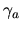for atoms and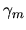for molecules. Any dependence on the charge state of the reflected particle is neglected, i.e., a finally negatively charged particle is assumed to produce as many secondary electrons as a finally neutral particle. Equations 3.2 to 3.6 show the transfered charge for each possibility considered in Figure 3.1. For the following calculation it is assumed thatis very close to one . The total current induced on the sample is then given by: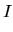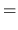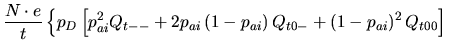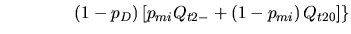(3.1)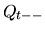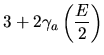(3.2)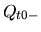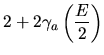(3.3)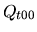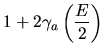(3.4)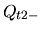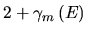(3.5)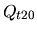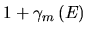(3.6)

• [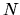]: Number of incident molecules
• [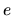]: Elementary charge
• [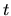]: Time
• [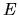]: Energy of primary molecules
• []: dissociation probability for an incident molecule
• []: probability for atoms to catch an electron
• []: probability for molecules to catch an electron
• [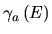]: secondary electron yield for incident atoms with energy• [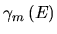]: secondary electron yield for incident molecules with energy• [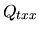]: net transfered charge as shown in Figure 3.1.
Assumingbeing very close to one, and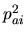small (typical values forare 0.02 to 0.2) the equation for the current simplifies to: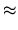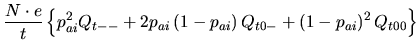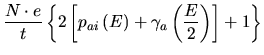(3.7)

We measured the secondary electron yield always at primary beam incidence angles of 30 and 60 degrees, respectively, as measured to the surface normal. At such steep angles the ionization efficiency, i.e, the fraction of negatively charged particles, drops considerably compared to angles used for ionization efficiency measurements (82 degrees). Considering a value forof 0.02 to 0.3 measured at grazing incidence and a rapid decrease towards steeper angles the influence ofwas neglected. The above equation thus simplifies to: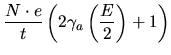(3.8)

To determine the secondary electron yield a measurement of the primary beam intensity has to be performed. This is done by biasing the sample to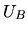= 18V with reference to ground. As the energy distribution for the secondary electrons peaks at a few eV virtually no secondary electrons escape from the sample and the measured current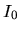is only caused by the incident primary beam (for the ionization efficiency the same simplifications as used above apply):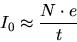(3.9)

The secondary electron yield for atomscan then be calculated using Equation 3.8 and 3.9: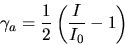(3.10)

Originally the secondary electron yield was determined by taking 6 measurements both for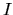and. From these measurements an average value forwas calculated. But this procedure was very time consuming and not reproducible very well mainly because an operator had to be present in front of the instrument. Any movement of the operator disturbed the current measurements in an unpredictable manner. Using an IEEE488 interface on the computer normally used to gather data for scattering measurements an automated measurement procedure was implemented as described below.

March 2001 - Martin Wieser, Physikalisches Institut, University of Berne, Switzerland